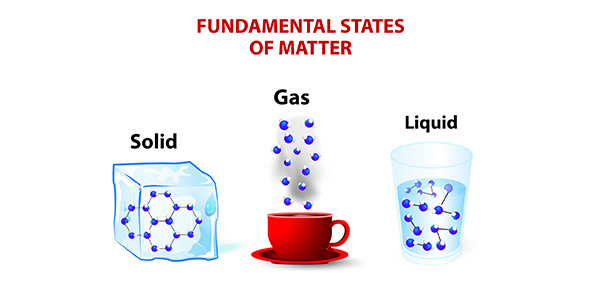# The Properties Of Matter

20 QuestionsSettingsIntroduction to Matter Chapter 1 Test

Related Topics
• 1.
Which property of matter is a measure of the gravitational force?
• A.

Density

• B.

Mass

• C.

Volume

• D.

Weight

• 2.
In a graduated cylinder containing several liquid layers, the least dense liquid is found
• A.

Floating at the top

• B.

In the middle layer

• C.

Settled at the bottom

• D.

Mixed throughout the cylinder

• 3.
How is a physical change different from a chemical change?
• A.

The change is reversible in a physical change

• B.

The change makes a new substance in a physical change

• C.

You get more volume in a physical change

• D.

Nothing happens in a physical change

• 4.
Melting candle wax would be an example of
• A.

Chemical change

• B.

Physical change

• C.

Mass change

• D.

Volume change

• 5.
Which of the following units would be best for describing the volume of mercury (liquid) used in an experiment?
• A.

Grams or kilograms

• B.

Meters or centimeters

• C.

Liters or milliliters

• D.

Newtons

• 6.
Which of the following is not a sign of a chemical change?
• A.

Change in color or odor

• B.

Change in state

• C.

Foaming or bubbling

• D.

Production of heat or light

• 7.
What chemical property is responsible for iron rusting?
• A.

Flammability

• B.

Conductivity

• C.

Reactivity with oxygen

• D.

Malleability

• 8.
To move a ball that has a mass of 150g would be more difficult than moving a ball with a mass of 50g because the ball with the mass of 150 g gas
• A.

Less weight than the 50g ball

• B.

Greater density than the 50g ball

• C.

More mass than the 50g ball

• D.

More volume than the 50g ball

• 9.
What unit of density would be appropriate to describe a solid bar of silver?
• A.

G/mL

• B.

G/cm

• C.

Oz/ft

• D.

Kg/L

• 10.
Which physical property of matter describes the relationship between mass and volume?
• A.

Ductility

• B.

Reactivity

• C.

Density

• D.

Weight

• 11.
Souring milk is an example of
• A.

Physical property

• B.

Physical change

• C.

Chemical property

• D.

Chemical change

• 12.
Malleability is an example of a
• A.

Physical property

• B.

Chemical property

• C.

Physical change

• D.

Chemical change

• 13.
Explain why volume and mass are not characteristic properties of matter, but density is.
• 14.
Why is density a useful property of identifying matter?
• 15.
After a tree is cut down with a chain saw, it is impossible to put the tiny wood chips back together.  The process cannot be reversed.  Does this mean that cutting a tree with a chainsaw causes a chemical change in the wood?  Explain why or why not.
• 16.
Summarize the differences between mass and weight.
• 17.
A graduated cylinder contains 4 liquids in 4 separate layers.  One liquid is water.  The purple liquid has a density of 1.62 g/cm .  The yellow liquid has a density of 0.46g/cm .  The red has a density of 0.91g/cm .  What is the order of the liquids in the cylinder bottom to top?  Explain your answer.  What would happen if you slipped a small chip of wood (0.85g/cm ) into the cylinder?
• 18.
Find the density of a substance that has a mass of 75g and a volume of 25cm .  Make sure you show your work and include ALL UNITS.
• 19.
Suppose you have a ball with a volume of 43cm .  You place the ball on a triple beam balance and find that it has a mass of 65g.  What would the density of the ball be?  Show work and label ALL UNITS.
• 20.
You place an unknown object into a graduated cylinder and it displaces 23mL of water.  you take the object out, dry it off, and place it on a triple beam balance.  You find that this unknown object has a mass of 20 g.  What would the density of the unknown object be? (Show work and ALL UNITS)  Would this unknown object float or sink in syrup (syrups density is 1.43g/cm )?